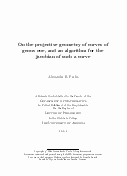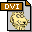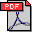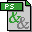# On the projective geometry of curves of genus one, and an algorithm for the jacobian of such a curve.
On the projective geometry of curves of genus one, and an algorithm for the jacobian of such a curve.
Ph.D. Dissertation, 2004.

Given equations with k-rational coefficients that define a curve C of genus 1 over a perfect field k, can we find equations that define its jacobian JC? The problem is trivial when the degree n of a k-rational divisor on C is equal to 1. For the cases 2<=n<=4, certain standard forms for C appear classically, and the classical invariant theory of those forms turns out to contain equations that define JC. This modern interpretation of classical results was explained for n=2 in 1954, for n=3 in 2001, and for n=4 in 1996. A standard form for C and its invariant theory was worked out by Tom Fisher for n=5 in 2003, again leading to equations for JC.

In the present work, the problem is solved algorithmically for all n>=3. (As in the classical approach, we must assume the characteristic of k does not divide n.) The basic idea, given to us by Minhyong Kim, is to embed C in Pkn-1 using the divisor of degree n, then to explicitly describe as matrices the finite Heisenberg group that corresponds to the n-torsion JC[n] on the jacobian, and then to determine equations for the quotient of C by the Heisenberg group, giving us the sought jacobian: C/JC[n]=JC. The Heisenberg matrices also allow us to compute the points of hyperosculation on C, which is a k-rational orbit under the action of JC[n] and thus gives the origin for the group law on JC. Our algorithm relies on techniques from the theory of Gröbner bases, and on techniques from the invariant theory of finite groups.

In presenting the background material to our algorithm, we develop the theory of curves of genus 1 with an attached k-rational divisor class, and the theory of non-degenerate degree n curves in Pkn-1 of genus 1. We thus state in a more general context results that appeared previously in more specialized contexts in work of Klaus Hulek and work of Catherine O'Neil. We give an elementary proof that the commutator pairing on the Heisenberg group corresponds to the Weil pairing on JC[n]. We describe intriguing hyperplane configurations and relate them to the points of hyperosculation on the curve of genus 1.On the projective geometry of curves of genus one, and an algorithm for the jacobian of such a curve.499K717K1.24MB